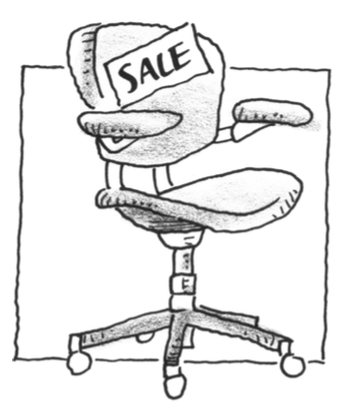### Home > APCALC > Chapter 12 > Lesson 12.3.1 > Problem12-98

12-98.

Multiple Choice: Acme office furniture is selling computer chairs for $145$ each and they are looking for ways to increase sales revenue. They know that companies have bought an average of $64$ chairs per order, so they decide to offer a lower price on larger orders. They are considering the following plan: the cost will be $145$ per chair for $80$ or fewer chairs, but for each chair over $80$ chairs, they will give a discount of $50¢$ per chair on the entire order. What is the maximum amount that any customer will have to pay for an order of chairs using this plan?

1. $17,112.50$

1. $13,912.50$

1. $21,312.50$

1. $145.00$

1. There is no maximum amount.Only consider orders $≥ 80$ chairs.

The cost of the chairs before the discount is $145x$ for $x$ chairs.

The discount for $81$ chairs is $0.50(1)(81)$.
The discount on $82$ chairs is $0.50(2)(82)$.
The discount on $83$ chairs is $0.50(3)(83)$.
$...$

The discount amount is $0.50(x - 80)x$.

Write an equation for the total cost, including the discount.
Then maximize the equation.
Remember, $x =\text{#}$ of chairs and the problem asks for the cost.